Lecture 23 - PHY294H

Example - A current ring

Find the magnetic field on the z-axis produced by a uniform circular current loop of radius R, which carries current I, and which lies in the x-y plane and is centered at the origin.

We shall integrate angle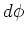over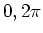in order to find the total B field. We take the current to be flowing counterclockwise around the loop. The magnetic field is along the z-axis, by symmetry. We take a position z on the z-axis and a small element of currenton the current loop. We take the angle between the vector from the loop to point z and the z-axis to be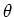. The cross productthen has magnitude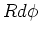that is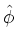and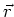are perpendicular. The component of Balong the z-axis is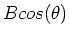putting this together, we find the magnetic field in the z-direction to be,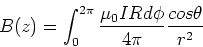(1)

From geometry, we have,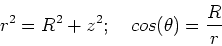(2)

The final result is then,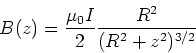(3)

Time varying electric and magnetic fields

The equations of electrostatics and magnetostatics are often summarized as Gauss' law for electrostatics,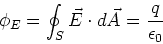(4)

Gauss' law for magnetostatics,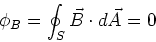(5)

Ampere's law for magnetostatics,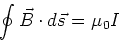(6)

To treat time varying fields, we are going to add another term to Ampere's law and we are going to introduce a new law called Faraday's law. The term which is added to Ampere's law was found by Maxwell in 1865. Faraday developed his law in 1831.

- Maxwell's term describes the fact that a time varying electric field induces a magnetic field.

- Faraday's law states that a time varying magnetic field induces an electric field.

These laws together describe the phenomena of electromagnetic induction. Faraday's law is more famous due to it many practical applications in motors, generators and other devices. The laws describing these time varying fields will also enable us to understand the propagation of light and other electromagnetic radiation. In that case we need both Faraday's law and Maxwell's term.

Maxwell's displacement current

Maxwell noticed that when a capacitor is charging, there is a logical inconsistency in Ampere's law. To understand this inconsistency, consider an initially uncharged capacitor connected to a voltage source at t=0. For simplicity, we consider a parallel plate capacitor. Current begins to flow in the circuit at t=0, charging up the capacitor. Now we can construct a loop around the wire in the circuit. However this loop does not really enclose the current in the wire. The loop can pass through the capacitor without cutting the wire. Therefor when the capacitor is charging, Amperes law would state that there is no enclosed current and hence the magnetic field is zero. This is wrong. There is a magnetic field produced by the current. Maxwell resolved this difficulty by adding a new term which includes the effect of the electric field which builds up between the capacitor plates. His idea was to related this electric field to the current flowing the circuit. From Gauss' law, we have,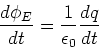(7)

or,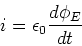(8)

This relates the current flowing into the capacitor to the electric field between the plates. Maxwell realized that if Amperes law is modified to,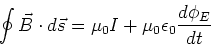(9)

then the logical inconsistency in the case of a charging capacitor is removed. This extra term is called the displacement current as it has the same dimensions as the true current in the circuit. His insight was brilliant as this equation is correct in general. Nevertheless this more general form of Ampere's law is still called Ampere's law! However Maxwell gets the last laugh as all four laws are collectively called Maxwell's equations as he was the first to really understand how they all work together to describe electromagnetism.

Example 30-7

A parallel plate capacitor is being charged at i = 1C/s. If the plates are circular with radius, R=0.1m, and are separated by d=1cm, find the magnetic field as a function of distance from the central axis of the capacitor.

Consider a circular loop or radius r centered on the axis of the capacitor and lying parallel to the plates. From Ampere's law we have,(10)

The electric flux through the loop is given by,(11)

so the rate of change of the electric flux is,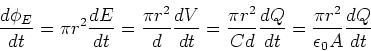(12)

Here we have used the relation for a capacitor Q=CVand the relation between electric field and voltage for a parallel plate capacitor E=V/d, and the expression for the capacitance of a parallel plate capacitor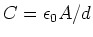. Evaluating the path integral for the magnetic field and equating it to the displacement current term, we then have,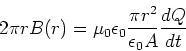(13)

or(14)